Friday, October 22, 2021
Home > CBSE Class 10 > Tangent to Circles CBSE Class 10 MCQ Questions with Answers Keys

# Tangent to Circles CBSE Class 10 MCQ Questions with Answers KeysHi students, Welcome to AMBiPi (Amans Maths Blogs). In this article, you will get Tangent to Circles CBSE Class 10 MCQ Questions with Answer Keys. You can download this PDF and save it in your mobile device or laptop etc.

Circles CBSE Class 10 MCQ Question No 41:

A, B, C, D are four distinct points on a circle whose center is O. If ∠OBD – ∠CDB = ∠CBD – ∠ODB, then find the measure of the angle A.

Option A : 45o

Option B : 60o

Option C : 120o

Option D : 135o

Option B : 60o

Circles CBSE Class 10 MCQ Question No 42:

PQ is a common chord of the two circles. APB is a secant line joining points A and B respectively on the two circles. Two equal tangents AC and BC are drawn. If ∠ACB = 45o, then find ∠AQB.

Option A : 75o

Option B : 90o

Option C : 120o

Option D : 135o

Option D : 135o

Circles CBSE Class 10 MCQ Question No 43:

ABCD is a cyclic quadrilateral. Tangents at A and C intersect at P. If ∠ABC = 100o, then find ∠APC.

Option A : 10o

Option B : 20o

Option C : 30o

Option D : 40o

Option B : 20o

Circles CBSE Class 10 MCQ Question No 44:

In the figure below, YAX is a tangent to the circle with center O. If ∠BAX = 70o and ∠BAQ = 40o, then find ∠ABQ.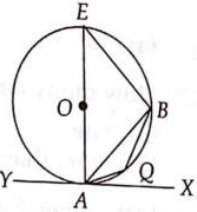Option A : 20o

Option B : 30o

Option C : 35o

Option D : 40o

Option B : 30o

Circles CBSE Class 10 MCQ Question No 45:

In the figure below, AP = 3 cm, PB = 5 cm, AQ = 2cm and QC = x cm. Find x.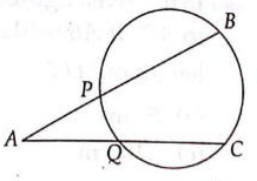Option A : 6 cm

Option B : 8 cm

Option C : 10 cm

Option D : 12 cm

Option D : None of these

Circles CBSE Class 10 MCQ Question No 46:

In the figure below, PT is a tangent to the circle with the radius 6 cm. If the distance between the point P to the center O is 10 cm. If PB = 5 cm, then find the length of chord BC.Option A : 7.8 cm

Option B : 8.2 cm

Option C : 8.4 cm

Option D : 9.3 cm

Option A : 7.8 cm

Circles CBSE Class 10 MCQ Question No 47:

In the figure below, O is the center of the circle. At the point T on the circle tangent UTV is drawn. If ∠VTR = 50o and triangle PTR is an isosceles triangle such that TP = TR, then find the sum (x + y + z).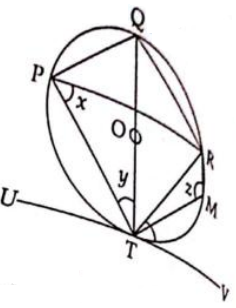Option A : 175 degree

Option B : 208 degree

Option C : 218 degree

Option D : 250 degree

Option C : 218 degree

Circles CBSE Class 10 MCQ Question No 48:

In the figure below, O is the center of the circle and if ∠AOD = 106o, then find ∠BCD.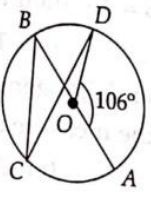Option A : 53o

Option B : 43o

Option C : 40o

Option D : 37o

Option D : 37o

Circles CBSE Class 10 MCQ Question No 49:

In the figure below, AB is the diameter of the circle and CD is perpendicular to AB. If AB = 10 cm and AE = 2 cm, then find DE.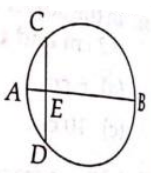Option A : 5 cm

Option B : 4 cm

Option C : √20 cm

Option D : √10 cm

Option B : 4 cm

Circles CBSE Class 10 MCQ Question No 50:

In the figure below, if ∠BAD = 60o, ∠ADC = 105o, then ∠DPC isOption A : 40o

Option B : 45o

Option C : 50o

Option D : 60o